# Carry Bit, Overflow Bit and Signed Integers

It has already been explained how the Carry Bit works for addition. Now there was interest in a comment about how it would work for negative numbers.

The point is, that the calculation of the carry bit does not have any dependency on the sign. The nature of the carry bit is that it is meant to be used for the less significant parts of the addition. So assuming we add two numbers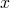and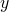that are having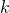andwords, respectively. We assume that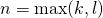and make sure thatandare both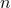words long by just providing the necessary number of 0-words in the most significant positions. Now the addition is performed as described by starting with a carry bit of 0 and adding with carry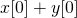, then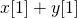and so on up to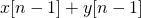, assuming thatis the least significant word and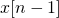the most significant word, respectively. Each addition includes the carry bit from the previous addition. Up to this point, it does not make any difference, if the numbers are signed or not.

Now for the last addition, we need to consider the question, if our result still fits inwords or if we need one more word. In the case of unsigned numbers we just look at the last carry bit. If it is 1, we just add one more word in the most significant position with the value of, otherwise we are already done withwords.

In case of signed integers, we should investigate what can possibly happen. The input for the last step is two signed words and possibly a carry bit from the previous addition. Assuming we have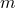-Bit-words, we are adding numbers between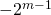and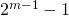plus an optional carry bit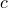. If the numbers have different signs, actually an overflow cannot occur and we can be sure that the final result fits in at mostwords.

If both are not-negative, the most significant bits ofandare both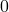. An overflow is happening, if and only if the sum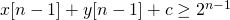, which means that the result „looks negative“, although both summands were not-negative. In this case another word with value 0 has to be provided for the most significant positionto express that the result is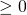while maintaining its already correctly calculated result. It cannot happen that real non-zero bits are going into this new most significant word. Consequently the carry bit can never become 1 in this last addition step.

If both are negative, the most significant bits ofandare both. An overflow is happening, if and only if the sum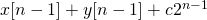, which means that the result „looks positive or 0“, although both summands were negative. In this case another word with valueor, depending on the viewpoint, has to be prepended as new most significant word. In this case of two negative summands the carry bit is always 1.

Now typical microprocessors provide an overflow flag (called „O“ or more often „V“) to deal with this. So the final addition can be left as it is inwords, if the overflow bit is 0. If it is 1, we have to signal an overflow or we can just provided one more word. Depending on the carry flag it isfor C=0 or all bits 1 (or, depending on the view point) for C=1.

The overflow flag can be calculated by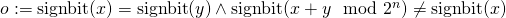.
There are other ways, but they lead to the same results with approximately the same or more effort.

The following table shows the possible combinations and examples for 8-Bit arithmetic and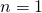:

x<0 or x≥0y<0 or y≥ 0(x+y)%2^8 < 0 or ≥ 0Overflow BitCarry Bitadditional word neededvalue additional wordExamples (8bit)
x≥0y≥0≥000no-0+0
63+64
x≥0y≥0<010yes064+64
127+127
x≥0y<0≥000 or 1no-65+(-1)
127+(-127)
x≥0y<0<000 or 1no-7+(-8)
127+(-128)
0+(-128)
x<0y≥0≥000 or 1no--9 + 12
-1 + 127
-127+127
x<0y≥0<000 or 1no--128+127
-128+0
-1 + 0
x<0y<0≥011yes-1-64 + (-65)
-128+(-128)
x<0y<0<001no--1 + (-1)
-1 + (-127)
-64 + (-64)

If you like, you can try out examples that include the carry bit and see that the concepts still work out as described.